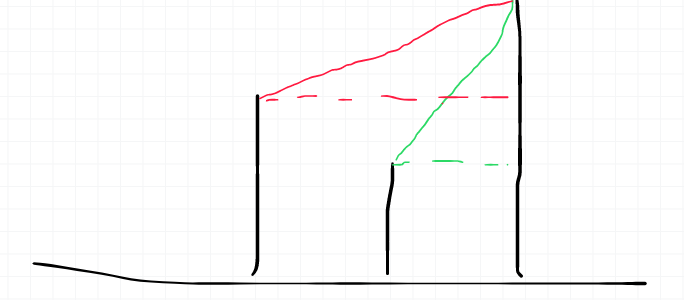# BZOJ1721 Ski Lift 缆车支柱

Description

Farmer Ron in Colorado is building a ski resort for his cows (though budget constraints dictate construction of just one ski lift). The lift will be constructed as a monorail and will connect a concrete support at the starting location to the support at the ending location via some number of intermediate supports, each of height 0 above its land. A straight-line segment of steel connects every pair of adjacent supports. For obvious reasons, each section of straight steel must lie above the ground at all points. Always frugal, FR wants to minimize the number of supports that he must build. He has surveyed the N (2 <= N <= 5,000) equal-sized plots of land the lift will traverse and recorded the integral height H (0 <= H <= 1,000,000,000) of each plot. Safety regulations require FR to build adjacent supports no more than K (1 <= K <= N - 1) units apart. The steel between each pair of supports is rigid and forms a straight line from one support to the next. Help FR compute the smallest number of supports required such that: each segment of steel lies entirely above (or just tangent to) each piece of ground, no two consecutive supports are more than K units apart horizontally, and a support resides both on the first plot of land and on the last plot of land.

Input

* Line 1: Two space-separate integers, N and K

* Lines 2..N+1: Line i+1 contains a single integer that is the height of plot i.

Output

* Line 1: A single integer equal to the fewest number of lift towers FR needs to build subject to the above constraints

Sample Input 1

13 4
0
1
0
2
4
6
8
6
8
8
9
11
12

Sample Output 1

5

Source

BZOJ-1721

dp[i]表示前i个至少需要修建几根柱子。dp[i]=max(dp[i] , dp[j] + 1)  ,  max(1,i-k) <= j <i && 斜率tmpk<=mink

 1 #include<bits/stdc++.h>
2 using namespace std;
3
4 double a;
5 int dp;
6 const int inf=0x3f3f3f3f;
7 double work(int x1,int x2,double y1,double y2) {
8     return (y1-y2)/(x1-x2);
9 }
10
11 int main() {
12     int n,k;
13     while(~scanf("%d%d",&n,&k)) {
14         for(int i=1;i<=n;i++) {
15             scanf("%lf",&a[i]);
16             dp[i]=inf;
17         }
18         dp=1;
19         for(int i=2;i<=n;i++) {
20             double mink=1e100;
21             for(int j=i-1;j>=max(i-k,1);j--) {
22                 double tmpk=work(i,j,a[i],a[j]);
23                 if(tmpk<=mink) {
24                     dp[i]=min(dp[i],dp[j]+1);
25                     mink=tmpk;
26                 }
27             }
28         }
29         printf("%d\n",dp[n]);
30     }
31 }

posted @ 2019-04-21 14:34  Frontierone  阅读(237)  评论(0编辑  收藏  举报